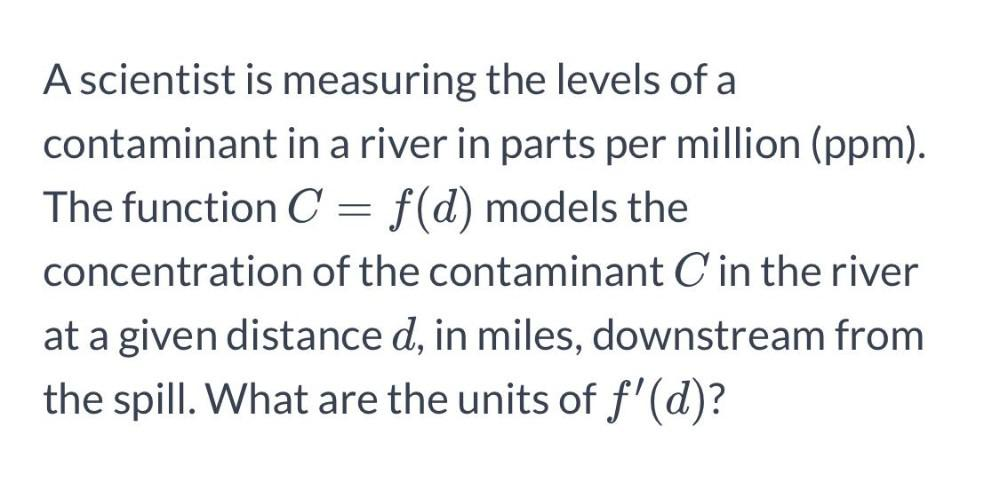Question:

# A scientist is measuring the levels of a contaminant in a river in parts per million (ppm). The function C = f(d) models the C cA scientist is measuring the levels of a contaminant in a river in parts per million (ppm). The function C = f(d) models the C concentration of the contaminant C in the river at a given distance d, in miles, downstream from the spill. What are the units of f'(d)?